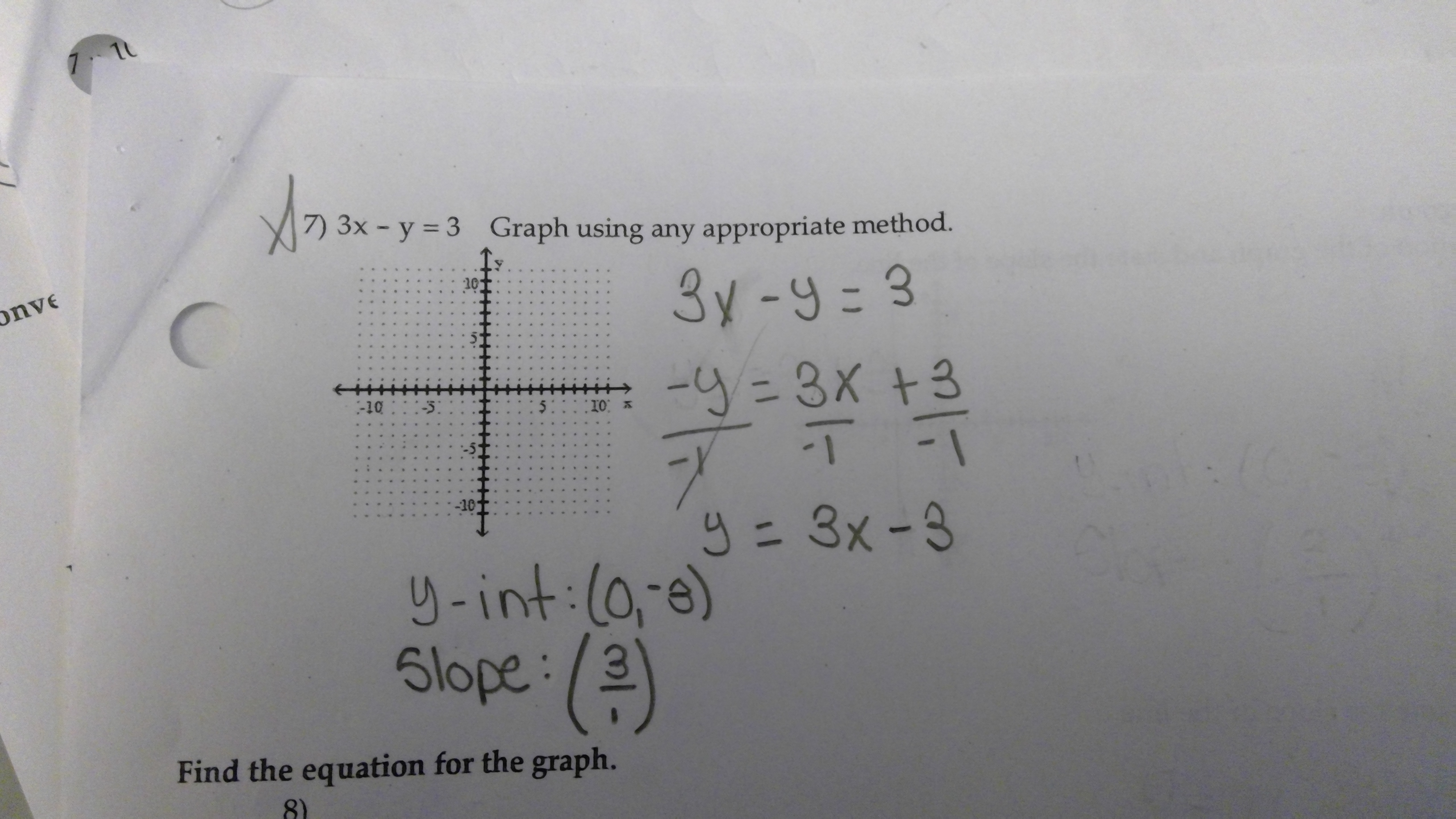# 7) 3x -y 3 Graph using any appropriate method. 10 Find the equation for the graph. 8)

Question

3x - y = 3 Graph using the appropriate method.

-y=3x+3

I divided everything by -1 so I can leave y by itself. How come 3x is not negative?help_outlineImage Transcriptionclose7) 3x -y 3 Graph using any appropriate method. 10 Find the equation for the graph. 8) fullscreen

### Want to see the step-by-step answer?